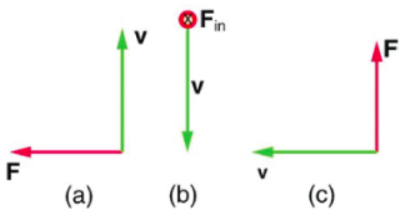# Problem: Consider the magnetic forces and particle velocities shown in the figurePart (a) What is the direction of the magnetic field that produces the magnetic force on a positive charge as shown in (a), assuming B is perpendicular to v?Part (b) What is the direction of the magnetic field that produces the magnetic force on a positive charge as shown in (b), assuming B is perpendicular to v?Part (c) What is the direction of the magnetic field that produces the magnetic force on a positive charge shown in (c), assuming B is perpendicular to v?

###### FREE Expert Solution

The magnetic force on moving charged particle:

$\overline{){\mathbf{F}}{\mathbf{=}}{\mathbf{q}}{\mathbf{\left(}}{\mathbf{v}}{\mathbf{×}}{\mathbf{B}}{\mathbf{\right)}}}$, where q is the charge of a particle, v is the velocity of the particle, and B is the magnetic field.

91% (191 ratings)###### Problem Details

Consider the magnetic forces and particle velocities shown in the figurePart (a) What is the direction of the magnetic field that produces the magnetic force on a positive charge as shown in (a), assuming B is perpendicular to v?

Part (b) What is the direction of the magnetic field that produces the magnetic force on a positive charge as shown in (b), assuming B is perpendicular to v?

Part (c) What is the direction of the magnetic field that produces the magnetic force on a positive charge shown in (c), assuming B is perpendicular to v?